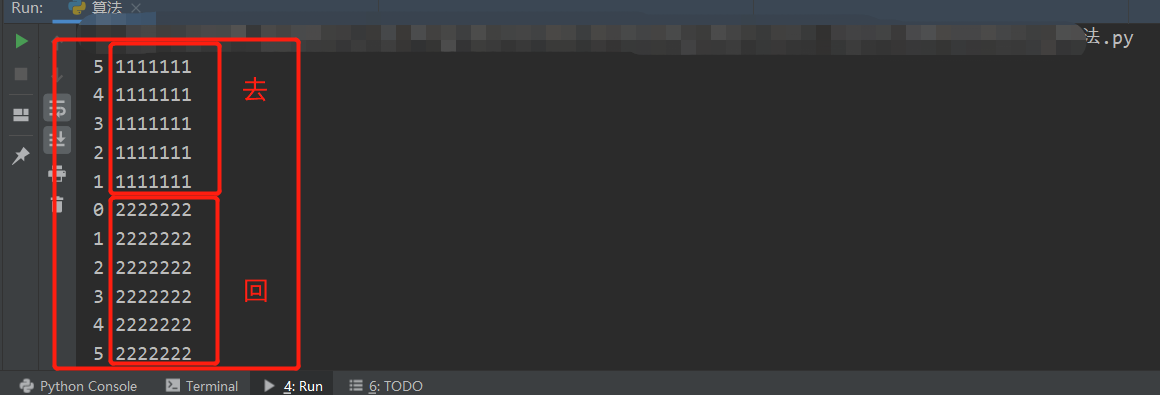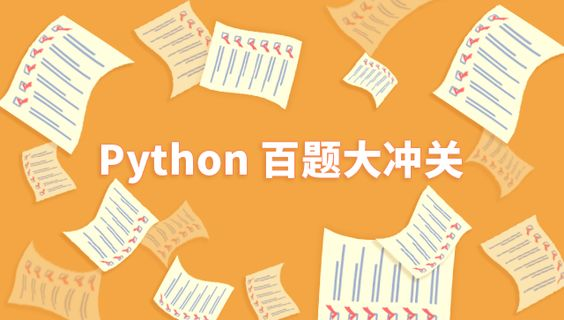• python算法题

千次阅读 2020-12-23 14:31:33
最近看了python的语法，但是总感觉不知道怎么使用它，还是先来敲敲一些简单的程序吧。1.题目：有1、2、3、4个数字，能组成多少个互不相同且无重复数字的三位数？都是多少？程序分析：可填在百位、十位、个位的数字都...

最近看了python的语法，但是总感觉不知道怎么使用它，还是先来敲敲一些简单的程序吧。

1.题目：有1、2、3、4个数字，能组成多少个互不相同且无重复数字的三位数？都是多少？

程序分析：可填在百位、十位、个位的数字都是1、2、3、4。组成所有的排列后再去掉不满足条件的排列。if __name__ == "__main__":

s = (1,2,3,4)

for a in s:

for b in s:

for c in s:

if a != b and b != c and c != a:

print "%d%d%d" %(a,b,c)2.题目：一个整数，它加上100后是一个完全平方数，再加上268又是一个完全平方数，请问该数是多少？

程序分析：在10万以内判断，先将该数加上100后再开方，再将该数加上268后再开方，如果开方后的结果满足如下条件，即是结果。请看具体分析：from math import sqrt

if __name__ == "__main__":

i = 1

while i < 100000:

a = int(sqrt(i + 100))

b = int(sqrt(i + 268))

if a **2 == (i + 100) and b **2 == (i + 268):

print i

i += 13.题目：输入某年某月某日，判断这一天是这一年的第几天？

程序分析：以3月5日为例，应该先把前两个月的加起来，然后再加上5天即本年的第几天，特殊情况，闰年且输入月份大于3时需考虑多加一天。if __name__ == "__main__":

days = {1:0,2:31,3:59,4:90,5:120,6:151,7:181,8:212,9:243,10:273,11:304,12:334}

year,month,day = input("year:"),input("month:"),input("day:")

if not days.has_key(month):

print "error input"

sum = days[month] + day

if month >= 2:

if year %400 == 0 or (year % 4 == 0 and year % 100 != 0):

sum += 1

print "it is the %dth day of the year." % sum4.题目：输入三个整数x,y,z，请把这三个数由小到大输出。

程序分析：我们想办法把最小的数放到x上，先将x与y进行比较，如果x>y则将x与y的值进行交换，然后再用x与z进行比较，如果x>z则将x与z的值进行交换，这样能使x最小。

if __name__ == "__main__":

a,b,c = input(),input(),input()

if a > b: a,b = b,a

if a > c: a,c = c,a

if b > c: b,c = c,b

print "small to big: %d,%d,%d" %(a,b,c)

或者：

if __name__ == "__main__":

s = [input(),input(),input()]

s.sort()

print "small to big: %s" %s

5.题目：输出9*9口诀。

程序分析：分行与列考虑，共9行9列，i控制行，j控制列。

if __name__ == "__main__":

for i in range(1,10):

for j in range(1,10):

print "%d*%d = %-3d" %(i,j,i*j),

print

6.题目：要求输出国际象棋棋盘。

程序分析：用i控制行，j来控制列，根据i+j的和的变化来控制输出黑方格，还是白方格。if __name__ == "__main__":

for i in range(8):

for j in range(8):

if (i + j) % 2 == 0:

print "%c"%219,else:

print "a",

print7.题目：古典问题：有一对兔子，从出生后第3个月起每个月都生一对兔子，小兔子长到第三个月后每个月又生一对兔子，假如兔子都不死，问每个月的兔子总数为多少？

程序分析： 兔子的规律为数列1,1,2,3,5,8,13,21....if __name__ == "__main__":

f1,f2 = 1,1

print "1: %d" %f1

print "2: %d" %f2

for i in range(3,21,2):

f1 = f1 + f2

print "%d: %d" %(i,f1)

f2 = f1 + f2

print "%d: %d" %(i+1,f2)8.题目：判断101-200之间有多少个素数，并输出所有素数。

程序分析：判断素数的方法：用一个数分别去除2到sqrt(这个数)，如果能被整除，则表明此数不是素数，反之是素数。from math import sqrt

if __name__ == "__main__":

count = 0

flag = 1

for a in range(101,201):

s = int(sqrt(a))

for i in range(2,s+1):

if a % i == 0:

flag = 0

break

if flag == 1:

count += 1

print a

else:

flag = 1

print "the total num is %d" %count9.题目：打印出所有的“水仙花数”，所谓“水仙花数”是指一个三位数，其各位数字立方和等于该数本身。例如：153是一个“水仙花数”，因为153=1的三次方＋5的三次方＋3的三次方。

程序分析：利用for循环控制100-999个数，每个数分解出个位，十位，百位。

if __name__ == "__main__":

print "water flower number is :",

for a in range(100,1000):

x,y,z = a/100,a/10%10,a%10

if x**3 + y**3 + z**3 == a:

print "%d,"%a,

10.题目：将一个正整数分解质因数。例如：输入90,打印出90=2*3*3*5。

程序分析：对n进行分解质因数，应先找到一个最小的质数k，然后按下述步骤完成：

(1)如果这个质数恰等于n，则说明分解质因数的过程已经结束，打印出即可。

(2)如果n<>k，但n能被k整除，则应打印出k的值，并用n除以k的商,作为新的正整数你n,

重复执行第一步。

(3)如果n不能被k整除，则用k+1作为k的值,重复执行第一步。if __name__ == "__main__":

n = input("please enter a number:")

print "%d = " %n,

for k in range(2,n+1):

while n != k:

if n % k == 0:

n = n/k

print "%d * " %k,

else:

break

print n11.题目：输入两个正整数m和n，求其最大公约数和最小公倍数。

程序分析：利用辗除法。if __name__ == "__main__":

s = [input(),input()]

s.sort()

a,b = s,s

while b != 0:

t = a % b

a = b

b = t

print "common divisor:%d"%a

print "common multiple:%d"%int(s*s/a)12.题目：求s=a+aa+aaa+aaaa+aa...a的值，其中a是一个数字。例如2+22+222+2222+22222(此时共有5个数相加)，几个数相加有键盘控制。

程序分析：关键是计算出每一项的值。if __name__ == "__main__":

print "please enter a and n:"

a,n = [input(),input()]

sum = a

if n > 1:

b = a

for i in range(2,n+1):

b = a + b*10

print b

sum += b

print "a+aa+aaa+... = %ld " %sum13.题目：一个数如果恰好等于它的因子之和，这个数就称为“完数”。例如6=1＋2＋3.编程找出1000以内的所有完数。

程序分析：因子就是所有可以整除这个数的数,不包括这个数自身。if __name__ == "__main__":

for n1 in range(3,1001):

sum = 1

n = n1

for k in range(2,n+1):

while n != k:

if n % k == 0:

n = n/k

sum += k

else:

break

sum += n

if sum == n1:

print sum14.题目：猴子吃桃问题：猴子第一天摘下若干个桃子，当即吃了一半，还不瘾，又多吃了一个，第二天早上又将剩下的桃子吃掉一半，又多吃了一个。以后每天早上都吃了前一天剩下的一半零一个。到第10天早上想再吃时，见只剩下一个桃子了。求第一天共摘了多少。

程序分析：采取逆向思维的方法，从后往前推断。

if __name__ == "__main__":

s1 = 1

for day in range(10,1,-1):

s2 = (s1+1)*2

s1 = s2

print "the total number is %d" %s1

15.题目：两个乒乓球队进行比赛，各出三人。甲队为a,b,c三人，乙队为x,y,z三人。已抽签决定比赛名单。有人向队员打听比赛的名单。a说他不和x比，c说他不和x,z比，请编程序找出三队赛手的名单。if __name__ == "__main__":

for x in ["a","b","c"]:

if x != "a" and x != "c":

print "x:%s"%x

for z in ["a","b","c"]:

if z != x and z != "c":

print "z:%s"%z

for y in ["a","b","c"]:

if y != x and y != z:

print "y:%s"%y16.题目：有一分数序列：2/1，3/2，5/3，8/5，13/8，21/13...求出这个数列的前20项之和。

程序分析：请抓住分子与分母的变化规律。if __name__ == "__main__":

a,b,s = 2,1,0

for n in range(1,21):

s += a/b

a = a+b

b = a-b

print "sum = %.3f" %s17.题目：求1+2!+3!+...+20!的和

程序分析：此程序只是把累加变成了累乘。if __name__ == "__main__":

a,s = 1,1

for n in range(2,21):

for i in range(1,n+1):

a *= i

s += a

a = 1

print "sum = %d" %s18.题目：利用递归方法求5!。

程序分析：递归公式：fn=fn_1*4!def fact(j):

if j == 0:

sum = 1

else:

sum = j * fact(j-1)

return sum

print fact(5)随笔分类 - Python学习

摘要: http://www.oschina.net/question/12_27127#INSTALLhttp://www.360doc.com/content/11/0606/23/54470_122122538.shtml照着以上的文章写了如下的代码，对于我这个不怎么写代码的人来说，敲出如下的代码好艰辛，有木有~~~~(>_

摘要: 最近看了python的语法，但是总感觉不知道怎么使用它，还是先来敲敲一些简单的程序吧。1.题目：有1、2、3、4个数字，能组成多少个互不相同且无重复数字的三位数？都是多少？ 程序分析：可填在百位、十位、个位的数字都是1、2、3、4。组成所有的排列后再去掉不满足条件的排列。if __name__ == "__main__": s = (1,2,3,4) for a in s: for b in s: for c in s: if a != b and b != c and c != a: ...阅读全文

摘要: 这里将介绍函数和对象--文件和流，让你在程序调用期间存储数据，并且可以处理来自其他程序的数据。一. 打开文件1.1 open函数open函数用来打开文件，语法如下：open(name,[.mode[.buffer]])它有唯一一个强制参数，就是文件名，然后返回一个文件对象。参数-模式和缓冲都是可选的。使用范例：(如果文件不存在，就会报错)>>> f = open('c:\\text\\test.txt') #windows下Traceback (most recent call last): File "", line 1, in f = 阅读全文

摘要: Python的标准安装包括一组模块，称为标准库。这里介绍模块的工作方式，学习如何使用它们。一. 模块1.1 用import从外部模块获取函数并为自己的程序所用：>>> from math import sqrt>>> import math1.2 编写自己的模块任何python程序都可以作为模块导入。程序保存的位置很重要。假设我们所写的程序叫hello.py被保存在c:/python目录下，可以执行以下的代码，告诉解释器在哪里寻找hello.py模块：#hello.pyprint "hello,world!">>> im阅读全文

摘要: 一. 迭代器在前面的笔记中，已经提到过迭代器(和可迭代)，这里会对此进行深入讨论。只讨论一个特殊方法---__iter__，这个方法是迭代器规则的基础。1.1 迭代器规则 迭代的意思是重复做一些事情很多次，到现在为止只是在for循环中对序列和字典进行迭代，但实际上也能对其他的对象进行迭代：实现__iter__方法的对象。 __iter__方法返回一个迭代器，所谓迭代器就是具有next方法(这个方法在调用时不需要任何参数)的对象。在调用next方法时，迭代器会返回它的下一个值。如果next方法被调用，但迭代器没有值可以返回，就会引发一个StopIteration异常。 为什么不用列表？列表是一次阅读全文

摘要: 在python中，有的名称会在前面和后面加上两个下划线，由这些名字组成的集合所包含的方法称为魔法方法(或者是特殊方法)。如果对象实现了这些方法中的某一个，那么这个方法会在特殊的情况下(确切地说是根据名字)被python调用。而几乎没有直接调用它们的必要。 这里会详细讨论一些重要的魔法方法(最重要的是__init__方法和一些处理对象访问的方法，这些方法允许你创建自己的序列或者是映射)，还会处理属性(通过property函数来处理)。一. 构造方法1.1 介绍与创建 首先要讨论的第一个魔法方法是构造方法，它代表着类似于以前例子中使用过的那种名为init的初始化方法。但构造方法和其他普通方法不同.阅读全文

摘要: Python被称为面向对象的语言，创建自己的对象是python非常核心的概念。这里会介绍如何创建对象，以及多态，封装，方法，特性，超类以及继承的概念。一. 对象的魔力面向对象程序设计中的术语 对象 基本上可以看做数据以及由一系列可以存取，操作这些数据的方法所组成的集合。对象最重要的几个有点包括以下几个方面：(1)多态：意味着可以对不同类的对象使用同样的操作，它们会像被施了魔法一样工作；(2)封装：对外部世界隐藏对象的工作细节；(3)继承：以普通的类为基础建立专门的类对象；1.1 多态 多态意味着就算不知道变量所引用的对象类型是什么，还是能对它进行操作，而它也会根据对象类型的不同而表现出不同的行阅读全文

摘要: 本文会介绍如何将语句组织成函数，还会详细介绍参数和作用域的概念，以及递归的概念及其在程序中的用途。一. 创建函数函数是可以调用，它执行某种行为并且返回一个值。用def语句即可定义一个函数：(并非所有的函数都会返回一些东西)def fibs(num): result = [0,1] for i in range(num-2): result.append(result[-2]+result[-1]) return result记录函数如果想给函数写文档以便让人理解的话，可以加入注释(以#开头)。另一个方式就是直接写上字符串，它会作为函数的一部分进行存储，这成为文档...阅读全文

摘要: 前面已经介绍过几种基本语句(print，import，赋值语句)，下面我们来介绍条件语句，循环语句。一. print和import的更多信息1.1 使用逗号输出A.打印多个表达式，用逗号隔开，会在每个参数之间插入一个空格符：>>> print 'age:',42age: 42B.同时输出文本和变量值，却又不希望使用字符串格式化：>>> name = 'Peter'>>> greeting = 'Hello'>>> print greeting,',',name阅读全文

摘要: 字典是python中唯一内建的映射类型。字典中的值并没有特殊的顺序，但是都存储在一个特定的键(key)里。键可以是数字，字符串甚至是元组。1. 创建和使用字典字典可以通过下面的方式创建：phonebook = {'Alice':'2341','Beth':'9102','Ceil':'3258'}字典由多个键及与其对应的值构成的对组成。每个键和它的值之间用冒号(:)隔开，项之间用逗号(,)隔开，而整个字典是由一对大括号括起来。空字典：{}1.1 dict函数可以用dict函数通过映射(比如其他字典)阅读全文

摘要: 这里会介绍如何使用字符串格式化其他的值，并了解一下利用字符串的分割，连接，搜索等方法能做些什么。1.基本字符串操作所有标准的序列操作(索引，分片，乘法，判断成员资格，求长度，取最大值和最小值)对字符串同样适用。但是要千万记住：字符串是不可变的。因此下面的分片赋值是不合法的：>>> website = "www.python.org">>> website[-3:] = "com"TypeError:object doesn't support slice assignment2.字符串格式化：精简版字符串格式化阅读全文

摘要: 最近在看《python基础教程》(基于python2.x)，下面总结一下第二章列表与元组的知识： 在这章中引入了数据结构的概念。数据结构是通过某种方式组织在一起的数据元素的集合。在python中，最基本的数据结构就是序列。序列中的每个元素被分配一个序号，即元素的位置，也被称为索引。注意：第一个索引是0。1.序列概览 python有6种内建的序列：列表，元组，字符串，Unicode字符串，buffer对象和xrange对象。 这里重点介绍列表和元组。列表和元组主要区别在于，列表可以修改，元组不可修改。一般来说，在几乎所有情况下列表都可以代替元组。 在需要操作一组数值的时候，序列很好用：Edwar阅读全文

摘要: 1.enumerate：返回2个值，1是当前的for循环的第几轮，2是循环得到的数值enumerate works by supplying a corresponding index to each element in the list that you pass it. Each time you go through the loop, index will be one greater, and item will be the next item in the sequence.choices = ['pizza', 'pasta', 'sa阅读全文

摘要: 关于string的split 和 join 方法对导入os模块进行os.path.splie()/os.path.join() 貌似是处理机制不一样，但是功能上一样。1.string.split(str=' ',num=string.count(str)): 以str为分隔，符切片string，如果num有指定值，则仅分隔num个子字符串。 S.split([sep [,maxsplit]]) -> 由字符串分割成的列表 返回一组使用分隔符(sep)分割字符串形成的列表。如果指定最大分割数，则在最大分割时结束。如果分隔符未指定或者为none，则分隔符默认为空格。注意：分隔阅读全文

摘要: print的即时打印会导致换行，要使得print的输出不换行，可以在字符串或者变量后面加个逗号(“,”)，如下：s = "A bird in the hand..."for c in s: if c.lower() == 'a': print "X", else: print c,阅读全文

摘要: list：创建：list = [5,7,9]取值和改值：list = list * 5列表尾插入：list.append(4)去掉第0个值并返回第0个值的数值：list.pop(0)去掉第0个值但不返回数值：del(list)去掉具体某个值：list.remove(35)函数function：无参数：def function():一个参数：def function(x):2个参数：def function(y):任意个参数：def add_function(*args):函数range：一个参数：range(n) 从第0位开始数n个位两个参数：range(m,n) 从第m位开阅读全文

摘要: 推荐一个学习语言的网站：http://www.codecademy.com有教程，可以边学边写，蛮不错的。for循环：1.for loops allow us to iterate through all of the elements in a list from the left-most (or zeroth element) to the right-most element. A sample loop would be structured as following:使用for循环可以遍历一个列表，从最左到最右：a = ["List of some sort”]for x阅读全文

展开全文• Python面试常见算法题集锦(递归部分) 1.用python写一个简单的递归函数 递归函数 : 自己调用自己的函数是递归函数 递:去 归:回 触发回的过程有2个条件: 回到上一层函数调用的位置 (1) 当前这层空间函数全部执行结束...

Python面试常见算法题集锦(递归部分)

1.用python写一个简单的递归函数

递归函数 : 自己调用自己的函数是递归函数
递:去
归:回

触发回的过程有2个条件: 回到上一层函数调用的位置
(1) 当前这层空间函数全部执行结束的时候,触底反弹,触发回的过程
(2) 遇到return 返回值, 直接返回到上一层空间
(3) 递归:  去的过程:就是不停的开辟空间,在回的时候,不停的释放空间,递归函数就是不停的开辟和释放空间的过程
回过程:最后一层空间所有代码执行完毕,会触发回的过程,或者遇到return返回值,也会触发回的过程,回到上一层函数调用的位置

注意事项:
1.递归每一层空间都是独立的个体,独立的副本,资源不共享,可以通过参数或者返回值形成共享
2.递归务必给予跳出的条件,如果递归的层数过深,不推荐使用.容易内存溢出或者蓝屏;

# 1.写一个简单的递归
def digui(n):
"""
打印1111,2222 是为了使读者看的更清楚 去 回  的两个过程区分
"""
if n > 0:
print(n, "1111111")
digui(n - 1)
print(n, "2222222")
digui(5)

运行结果如下图：2.求任意数n的阶乘 5! 5x4x3x2x1=?

def jiecheng(n):
if n<=1:
return 1
return n*jiecheng(n-1)
res = jiecheng(5)
print(res)

# 代码解析:
# 去的过程
n = 5 return n*jiecheng(n-1) => 5*jiecheng(4)
n = 4 return n*jiecheng(n-1) => 4*jiecheng(3)
n = 3 return n*jiecheng(n-1) => 3*jiecheng(2)
n = 2 return n*jiecheng(n-1) => 2*jiecheng(1)
n = 1 return 1

# 回的过程
n = 2 return n*jiecheng(n-1) => 2*1
n = 3 return n*jiecheng(n-1) => 3*2*1
n = 4 return n*jiecheng(n-1) => 4*3*2*1
n = 5 return n*jiecheng(n-1) => 5*4*3*2*1

5*4*3*2*1 = 120

3.斐波那契数列: 1,1,2,3,5,8,13,21,34,55 ,… 第n个数字是几?

def fbnq(n):
if n == 1 or n == 2:
return 1
return fbnq(n - 1) + fbnq(n - 2)

res = fbnq(5)
print(res)

# 代码解析
斐波那契数列简单地说，起始两项为01，此后的项分别为它的前两项之和
F（0=0，F（1=1，F（n）=F(n-1)+F(n-2)（n≥2，n∈N*）

fbnq(5) = fbnq(4) + fbnq(3) = fbnq(3) + fbnq(2) + fbnq(3) => fbnq(2) + fbnq(1) + fbnq(2) + fbnq(2) + fbnq(1)
=> 1+1+1+1+1 = 5

4.青蛙跳台阶: 一只青蛙要跳上n层高的台阶,一次能跳一级,也可以跳两级,请问这只青蛙有多少种跳上这个n层高台阶的方法?

青蛙

def Frog_JumpSteps_digui(n):
if n in (1, 2):
return 1
return Frog_JumpSteps_digui(n - 1) + Frog_JumpSteps_digui(n - 2)

res = Frog_JumpSteps_digui(2)
print(res)

代码解析
可以转化为斐波那契数列的方式进行求解，假设要跳N阶台阶，那么第一步有两种跳法：
（1）跳一步，后面还有n-1个台阶需要跳；
（2）跳两步，后面还有n-2个台阶需要跳。
可以看到跳n阶台阶的跳法数等于跳n-1和n-2阶台阶数的和，即F(n) = F(n-1) + F(n-2)

展开全文python 算法
• 看书，看视频都可以帮助你学习代码，但都只是辅助作用，学好 Python，最重要的...Python大冲关​www.shiyanlou.com以下是前四道题目：一、确定字符串是否包含唯一字符题目介绍：实现一个算法：识别一个字符串...

看书，看视频都可以帮助你学习代码，但都只是辅助作用，学好 Python，最重要的还是 多敲代码，多刷题。

不知道怎么找题刷题的小伙伴，可以看看我们今天带来的覆盖了基本语法，数据结构，算法等多个方面，并附有答案。

100 道 Python 练习题，刷起来！！！Python 百题大冲关​www.shiyanlou.com以下是前四道题目：

一、确定字符串是否包含唯一字符

题目介绍：

实现一个算法：识别一个字符串中，是否包含唯一的字符。如果字符串中的字符都是唯一的，则返回 True，如 '123'。

如果字符串中的字符有重复，则返回 False，如 '1223'。

考核点：集合的用法：set()

解题思路：

看到这个题目，你的第一反应可能是：先把字符串中每个字符都拆分出来，放入一个列表，再统计列表中是否有数量为 1 的字符。但这么做不但费时，而且要循环多次，效率极低，有一个方法可以让你超过 99 % 的面试者：

记得 Python 的 Set (集合) 概念吗？在集合中，所有的元素都是唯一的。你可以使用 set() 方法将字符串、列表等任何「可迭代对象」转换为集合，转换后会删除重复的元素，继而长度会发生变化。

所以，我们只需要将字符串转换为集合，然后比较转换前后的长度即可，代码如下。

参考答案：

class UniqueChars(object):

def has_unique_chars(self, string):

if string is None:

return False

return len(set(string)) == len(string)

二、确定字符串是否是另一个的排列

题目介绍：

实现一个算法来识别一个字符串 str2 是否是另一个字符串 str1 的排列。排列的解释如下：如果将 str1 的字符拆分开，重新排列后再拼接起来，能够得到 str2 ，那么就说字符串 str2 是字符串 str1 的排列。例如：123 是 321 的排列，abc 是 cba 的排列。

如果 str2 字符串是 str1 字符串的排列，则返回 True；反之则返回 False；

考核点：sorted()方法

解题思路：

一样是看起来复杂，但只需一行核心代码就能搞定的题目。在其他语言中，你可能要手动写一个排序算法，然后比较两个字符串排序后是否一样。但 Python 自带的 sorted() 方法，可以自动对所有可迭代的对象进行排序操作。

参考答案：

class Permutations(object):

def is_permutation(self, str1, str2):

if str1 is None or str2 is None:

return False

return sorted(str1) == sorted(str2)

附加知识点：

sort() 和 sorted() 的区别：sort() 应用在 list 列表中，而 sorted() 可以对所有可迭代的对象进行排序操作。

三、反转字符串中的字符

题目介绍：

实现一个算法来实现反转字符数组的功能。反转的要求如下：将字符数组的字符进行反转，例如 ['b', ' ', 'a', 'r'] 变成 ['r', 'a', ' ', 'b']。

将字符数组替换为反转后的数组。

考核点：使用 a,b = b,a 交换变量

解题思路：

常规的解法是创建一个相同长度的新数组，然后把第一个数组中的元素，按倒序放入新数组中。但更简单的方法是：利用 Python 交换变量的特性，不需要新建数组，直接在原数组里即可完成，代码如下。

参考答案：

class ReverseString(object):

def reverse(self, chars):

if chars:

size = len(chars)

for i in range(size // 2):

chars[i], chars[size - 1 - i] = \

chars[size - 1 - i], chars[i]

return chars

四、Fizz Buzz 经典问题

题目介绍：

给定一个整数 num，从 1 到 num 按照下面的规则返回每个数：如果这个数被 3 整除，返回 'Fizz'。

如果这个数被 5 整除，返回 'Buzz'。

如果这个数能同时被 3 和 5 整除，返回 'FizzBuzz'。

如果这个数既不能被 3 也不能被 5 整除，返回这个数字的字符串格式。

考核点：Python 基础语法中的条件判断

解题思路：

有趣又比较简单的一道题，写一个循环即可。当数字被 3 整除时返回 'Fizz'，被 5 整除，返回 'Buzz'，同时被 3 和 5 整除，返回 'FizzBuzz'……代码如下。

参考答案：

class Solution(object):

def fizz_buzz(self, num):

if num is None:

raise TypeError('num cannot be None')

if num < 1:

raise ValueError('num cannot be less than one')

results = []

for i in range(1, num + 1):

if i % 3 == 0 and i % 5 == 0:

results.append('FizzBuzz')

elif i % 3 == 0:

results.append('Fizz')

elif i % 5 == 0:

results.append('Buzz')

else:

results.append(str(i))

return results

更多题目，欢迎进入课程学习～新用户可以免费学习前 20 道题目！Python 百题大冲关​www.shiyanlou.com展开全文• 不知对错，大一的简单算法题（零比特）
#零比特填充
while True:
try:
str1 = []
num = []
s = input()
for x in s:
str1.append(x)
j = 0
for i in range(len(s)):
if (str1[i] == '1'):
j = j + 1
else:
j = 0
if (j == 5):
num.append(i + 1)
# print("i:", i, "j:", j)
j = 0
k = 0
for i in range(len(num)):
str1.insert(num[i] + k, '0')
k = k + 1
# print(str1)
str2 = "".join(str1)
print(str2)
except EOFError:
break

展开全文算法 python 开发语言
• = 0: print('{0}是闰年'.format(n)) else: print('{0}不是闰年'.format(n)) 高效方法： python 的 calendar 库中已经封装好了一个方法 isleap() 来实现这个判断是否为闰年： import calendar n = int(input("请...python做算法题
• Python 算法题之 俄罗斯套娃信封 文章目录Python 算法题之 俄罗斯套娃信封给出题目 展示一个错误示例动态规划参考资料相关博客 给出题目 有一个二维整数数组 envelopes ，其中 envelopes 中的每个值代表着一个信封...算法 leetcode 动态规划 Python
• 下面向大家介绍几个python算法题。一：二分法求平方根1.题目要求为2.输入输出格式为3.博主解题的思路这道题在c语言中是一道经典的题目，可以用循环，或者递归，在这里我们用python来写。无论是循环还是递归，都是...
• 写在前面最近也没什么事可做,就在备赛蓝桥杯(Python).蓝桥杯主要考察的是算法题目.所以我也在网上找了些资源刷题,昨天当我刷到《完美的代价》这道题目的时候,我就被卡住了.怎么想也想不通,就连解题代码也看不懂.更...
• python算法题 python123网站单元四题目 目录 一:二分法求平方根 二:Collatz猜想 三:算24(只考虑满足,不考虑把所有情况找出来)   下面向大家介绍几个python算法题. 一:二分法求平方根 1.题目要求为 2.输入输出格式...
• 原标题：给大家分享一个「Python算法题」分苹果今天刷到一道算法题，分享一下果园里有堆苹果，N(1)只熊来分。第一只熊把这堆苹果平均分为N份，多了一个，它把多的一个扔了，拿走了一份。第二只熊把剩下的苹果又平均...
• 一：基础算法题5道1.阿姆斯特朗数如果一个n位正整数等于其各位数字的n次方之和,则称该数为阿姆斯特朗数。判断用户输入的数字是否为阿姆斯特朗数。(1)题目分析：这里要先得到该数是多少位的，然后再把每一位的数字...
• 递推法def eat01(n=10): x = 1 while n > 1: # 因为从10开始循环，第十天是1个桃，终止条件不能等1，否则就多算了1天 x = (2 * x + 2) n -= 1 return x 这道用递归的方法也能解决。 上面的公式...
• 各种类型的算法import copy# def num():# return [lambda x, i=i: x*i for i in range(4)]# print([m(2) for m in num()])# M(2)是取值得2倍 这个问题涉及到了Python的闭包及延时绑定的知识(Python作用域)。...
• 一些自己在面试自动化测试或测试开发岗时常被问到的python算法题，大多都是一些列表相关的操作，在此记录并和大家分享，转载请注明出处。 1.写一个函数比较两个列表是否相等 a=[1,2,3] b=[2,3,1] c=[1,4,3] a与b为...
• leetcode-python-合并两个有序数组今天第一次电话面试，有点紧张，所以自己感觉表现的不是很好，面试过程中面试官问到了两个算法题，都是leecode上面的题目，而且我都做过，但是当时一下子都想不起来，回答的方法也...
• 题目重现：这道和三数之和其实很像了，一个枚举其余的使用指针。经过我改造后的程序如下：classSolution:deffourSum(self,nums,target):n=len(nums)nums.sort()ans=list()ifnnums[-1]*4:return[]forainrange(n):if...
• 比如有这么到,题目是:您住在笛卡尔市，那里的所有道路都以完美的网格布局。 您提前十分钟到达预约地点，所以您决定趁机散步。 该城市在其手机上为其市民提供了一个“步行生成”应用程序-每次...
• Python 经典算法100及解析(小结) 1：找出字符串s=”aaabbbccceeefff111144444″中，字符出现次数最多的字符 （1）考虑去重，首先将字符串进行过滤去重，这样在根据这些字符进行循环查询时，将会减少循环次数，提升...python 算法 自然语言处理
• #HJ1 计算字符串最后一个单词的长度，单词以空格隔开。 """ word = input("请输入单词，并以空格间断:") new_word = word.split(' ') print(len(new_word[-1])) """ #HJ2 写出一个程序，接受一个由字母和数字组成的...
• 同一行中输入两个（多个）值 比如输入n， m两个数字 n, m = map(int, input().split()) 一行一行输入n行m列的矩阵 for i in range(n): a = str(input()) a = a.split(" ") a = list(a) a = map(int, a) ...
• 这道核心在于如何使得左子树的右孩子指向右子树的左孩子，因为这两个节点之间不是同一个父节点 非递归解法，使用广度优先遍历，也就是层序遍历，在遍历每层的时候为next赋值 #使用广度优先遍历，广度优先遍历的...二叉树 算法 python
• 问题1：编写一个程序，找到2000年至3200年(包括在内)中所有可被7整除但不能被5整除的所有数字，得到的数字按逗号分隔，打印在一行上。 # method1 for i in range(2000, 3201): if i % 7 == 0 and i % 5 !...python 算法 开发语言
• Python算法题----孙悟空吃蟠桃，孙悟空偷蟠桃的故事, 孙悟空第一天摘下孙悟空第一天摘下若干蟠桃，当即吃了一半，还不过瘾，又多吃了一个。第二天早上，他又将剩下的蟠桃吃掉了一半，还不过瘾，又多吃了一个。之后...
• 第1章 算法初步 1n1．1　什么是算法　1n1．1．1　算法的定义　1n1．1．2　算法与程序的...3n1．2．4　时间复杂度的意义　6n1．3　空间复杂度　8n1．4　算法的应用　8n1．5　Python算法的优势　9n1．6　小结　9n1．7...
• def COMB(x): # 动态规划求组合数，也可以用python自带的函数求，math.comb lst = [[1 for _ in range(x+1)] for _ in range(x+1)] for i in range(2, x+1): for j in range(1, i): lst[i][j] = lst[i-1][j] + lst[i...算法 python 开发语言...

python算法题python 订阅